Equable Rectangular Pentagon

EQUABLE RECTANGULAR PENTAGON
and related topics

Balmoral Software

Solutions: 3

### Solutions

We define a "rectangular pentagon" as a house-shaped, integer-sided convex plane figure consisting of a triangle with base B and sides u and v, surmounting a rectangle of width B and height d: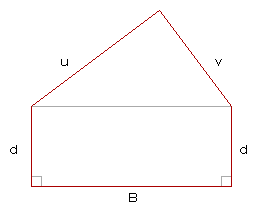A rectangular pentagon can be thought of as an integer-sided convex pentagon with the added restriction that two non-adjacent sides have equal lengths, each of which forms a right angle with the intervening side.

The notation (Base,Side,Side,Height) = (B,u,v,d) will be used to describe the rectangular pentagon. The sides u and v of the triangular portion can be swapped without changing the area or perimeter of the pentagon, so we won't consider these to be separate solutions and can take u ≥ v.

There are exactly 3 equable rectangular pentagons.

Proof. For a convex rectangular pentagon, the base angles of the triangular portion must be acute. Thus, if the triangle is obtuse or right, then only its longest side can be the base.

By convention, the area of an equable rectangular pentagon is an integer, as is that of its rectangular portion, so by subtraction the triangle is Heronian. For an equable rectangular pentagon, we have

 A = AT + Bd = PT + 2d = P, 
where AT and PT are the integer area and integer perimeter of the triangular portion. Since each side of a Heronian triangle must be at least 3, we have B ≥ 3 and can solve  for d: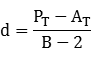Since d ≥ 1, we have
PT - AT ≥ B - 2 ≥ 1,
so the triangle is perimeter-dominant, and we can write
 B ≤ PT - AT + 2 
Referring to results on Heronian perimeter-dominant triangles, we now consider each of these triangles as a candidate for the upper portion of an equable rectangular pentagon.

Case 1: (6,5,5) triangle

PT = 16
AT = 12
By , a solution for d can exist only when
B ≤ 16 - 12 + 2 = 6,
which is satisfied when any of the triangle sides is the base. However, by , only a base of 6 results in an integer height d:
Bd
64/(6 - 2) = 1
54/(5 - 2) = 4/3
The single result is the (6,5,5,1) rectangular pentagon.

Case 2: (8,5,5) triangle

PT = 18
AT = 12
Since this triangle is obtuse, only the side of length 8 can be the base, and by ,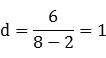The single result is the (8,5,5,1) rectangular pentagon.

Case 3a: (a,a-1,3) triangles, a ≥ 3

By results presented elsewhere, these triangles are not acute, so by , we have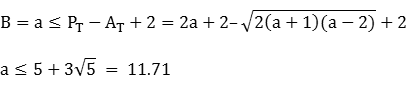The only feasible solution in the Case 3a results table is the (5,4,3) triangle with B = a = 5, PT = 12 and AT = 6, so by ,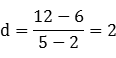The single result is the (5,4,3,2) rectangular pentagon.

Case 3b: (a,a-2,4) triangles, a ≥ 4

Again, these triangles are not acute, so by , we have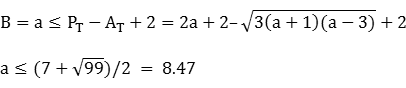The only feasible solution in the Case 3b results table again is the (5,4,3) triangle.

### Summary

Summary of equable rectangular pentagons:

Base,side,side,heightPerimeter=Area
(5,4,3,2)16
(6,5,5,1)18
(8,5,5,1)20### Solid of revolution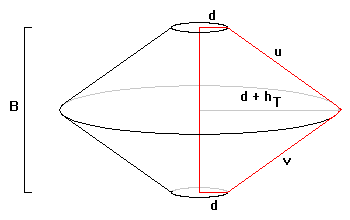One of the three equable rectangular pentagons produces an equable solid of revolution by rotating it around its base. Let hT be the height of the triangular portion of the pentagon, so that
hT = 2AT/B
Then the solid of revolution is two back-to-back conical frustums, each defined by radii d and d + hT. One frustum has a slant height of u and the other a slant height of v. The corresponding frustum heights sum to B. The total volume and surface area of the solid of revolution are V and S, where
V/π = B[d2 + d(d + hT) + (d + hT)2]/3 = d2B + 2dAT + 4AT2/(3B)

S/π = (d + d + hT)(u + v) + 2d2 = 2(d + AT/B)(u + v) + 2d2

Evaluating these formulas for the three equable rectangular pentagons, the (6,5,5,1) one gives rise to an equable solid with V = S = 62π:
BuvdATVS
54326268π/5264π/5
65511262π62π
85511256π52π

### Cyclic rectangular pentagons

In this section, we explore the infinitely-many integer-sided rectangular pentagons that are cyclic: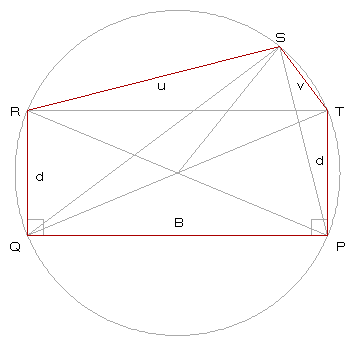B, u, v and d are positive integers.

The four corners of the rectangular portion of a cyclic rectangular pentagon (B,u,v,d) are co-circular, so the pentagon's circumcenter is the centroid of the rectangle, which is the intersection of the two diagonals of the rectangle. Therefore, the ascending diagonal QT of the rectangle is a diameter D of the circumcircle, which forms a right triangle PQT with the base B and the right side d:

 B2 + d2 = D2 
Since B and d are integers, so is D2.

Consider the two radii of length D/2 connecting the circumcenter to the endpoints R and S of the upper-left side u. By the Triangle Inequality,

v ≤ u < D/2 + D/2 = D
Next, consider the cyclic quadrilateral QRST that is contained in a semicircle. Since QST is a right triangle, the diagonals of this quadrilateral are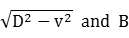Applying Ptolemy's Theorem, we haveUsing , this reduces to
 D4 - (d2 + u2 + v2)D2 = 2duvD  D3 - (d2 + u2 + v2)D - 2duv = 0 
Since D2 is an integer, the left side of  is an integer, and so D is rational. It follows that D is an integer.

At this point, we can establish that none of the three equable rectangular pentagons satisfies , so they are not cyclic.

The circumcircle of triangle RST is the same as that of the aforementioned quadrilateral. By the SSS Theorem, the circumradius D/2 is related to the triangle area AT by:so AT is rational. By , the cyclic rectangular pentagon has rational area, and so it is a Robbins pentagon. It follows from Lemma 5 of [A] that the rectangular pentagon area is an integer, and triangle RST is therefore Heronian. By Theorem 8 of [A], all of the diagonals of the cyclic rectangular pentagon are rational since three of them are the integer diameter D and the upper triangle base B. It follows that the remaining diagonals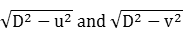are rational, and by the previous reasoning, are integers. Therefore, the area and diagonals of a cyclic integer-sided rectangular pentagon are all integers.

Cyclic rectangular pentagons may be found by searching over the following domain:

• 1 ≤ B ≤ some limit
• 1 ≤ d ≤ some limit
• D2 = B2 + d2, where D is a hypotenuse number (OEIS A009003)
• 1 ≤ v ≤ u < D
• If  is satisfied, then (B,u,v,d) is a cyclic rectangular pentagon
By , the area of a cyclic rectangular pentagon is
A = B[d + uv/(2D)]
The height of the pentagon is
h = d + 2AT/B = d + uv/D,
so its height/width aspect ratio is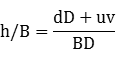Following is a list of the 22 cyclic rectangular pentagons with sides not exceeding 100, sorted by diameter and area:
BuvdCircumcircle
diameter D
AreaPerimeterP-S
diagonal
Q-S
diagonal
241515725276682020
201571525342722024
483030145011041364040
403014305013681444048
635225166516381723960
605216256518841783963
603933256520941825256
392516526521481846063
563925336522681865260
523325396523581885660
724545217524842046060
604521457530782166072
846840138524362185175
756813408533902365184
514013688536242407584
775140368536962406875
755136408538102426877
684036518540442467577
9660602810044162728080
8060286010054722888096
7544351001257962354117120
1007535751258550360100120
The corresponding diagrams can be viewed here.

The 889 cyclic rectangular pentagons with sides not exceeding 1000 are listed here, sorted by diameter and area.

### Reference

[A] Buchholz, Ralph H. & MacDougall, James A. (2008), "Cyclic polygons with rational sides and area", Journal of Number Theory, 128 (1): 17-48.